## Mensuration

### Methods to Find Area of Quadrilaterals and Polygons:

We can find the area of the triangle using the formula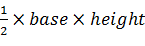To find the area of a quadrilateral we will divide the quadrilateral into two triangles and add the areas of the two triangles.

#### Triangulation

The method of dividing a quadrilateral into two triangles to find out its area is known as triangulation.

Let ABCD is a quadrilateral. Then
= (Area of ∆ ABD) + (Area of ∆ BCD)
= ½ d (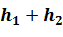), where d is the diagonal and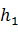and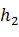are the heights of the quadrilateral.
Area of quadrilateral ABCD= ½ d (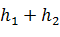)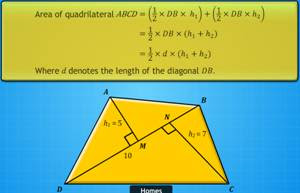In a parallelogram the diagonal divide it into two triangles. Now

Area of a Parallelogram = base × height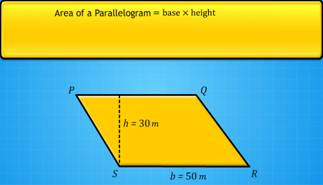### Rhombus

In a rhombus diagonals are perpendicular to each other, so we can use the method of triangulation to find the area of a rhombus
Area of a Rhombus =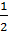(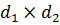), where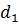and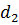are the lengths of the diagonals.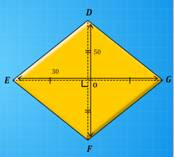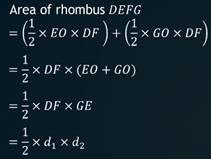Area of a Rhombus =(), whereandare the lengths of the diagonals.

### Trapezium

#### A trapezium has a pair of parallel sides.

Area of trapezium =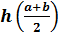Where h = perpendicular distance between the parallel sides of a trapezium and a and b are the lengths of the parallel sides.
Area of trapezium =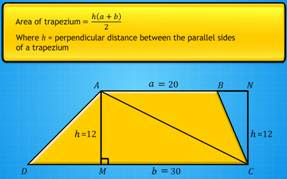### Polygon

polygon is a closed shape that has at least three sides. It is a closed shape that has at least three sides. Triangles, quadrilaterals, rectangles and squares are all types of polygons. Moreover, a polygon can be of any shape and can have any number of sides. There is no specific formula for calculating the area of a polygon. The best way is to split the polygon into shapes whose area can be calculated individually.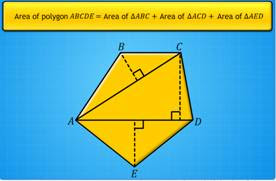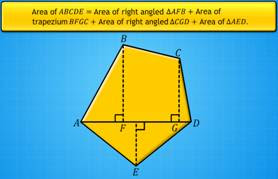### Surface Area of Solids

Cube: A cube is a three-dimensional figure, a solid made up of six equal squares called faces.

The lateral surface area (LSA) of a cube is equal to 4l2, whereas its total surface area (TSA) is 6l2.

Cuboid: A cuboid is a solid made up of six rectangles, called faces. The total area of a cuboid is the sum of the areas of these faces.
The lateral surface area (LSA) of a cuboid is 2h (l + b), whereas its total surface area (TSA) is (lb + bh + hl).

Cylinder:
A cylinder is a solid composed of two congruent circles in parallel planes.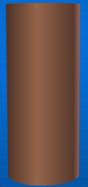The curved surface area (CSA) of a cylinderical pillar is 2πrh , whereas its total surface area (TSA) is  2πr(r+ h).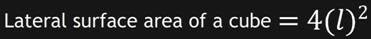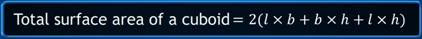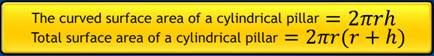### Volume of Solids

Bodies that occupy space are called solids.
Solid bodies occur in various shapes, such as cuboid, cube, cylinder and cone.
The space occupied by a solid body is called its volume.
The units for volume are cubic centimetre(cm3), cubic metre(m3) etc.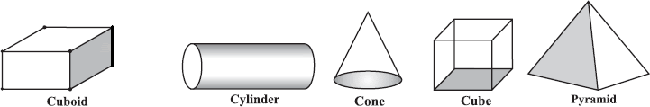A cuboid is a solid bounded by six rectangular plane faces.
Consider a cuboid of length, breadth and height , and , respectively. Then:
• Volume of cuboid = lbh cubic units
• Total surface area of cuboid = 2(lb + bh + lh)sq.units
• Lateral surface area of cuboid = Area of 4 walls = Area of 4 walls = 2h(l + b)sq.units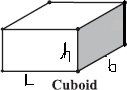A cube whose length, breadth and height are all equal is called a cube.
Consider a cube of edge a units. Then:
• Volume of cube = a3 cubic units
• Total surface area of cube = 6a2 sq.units
• Lateral surface area of cube = 4a2 sq.units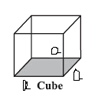A solid bounded by a cylindrical surface and two parallel circular bases at the top and bottom is called a cylinder.
Consider a cylinder of radius r units and height h units. Then:
• Volume of cylinder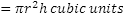• Total surface area of cylinder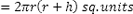• Lateral surface area of cylinderThe volume of a cylinder is also sometimes known as its capacity.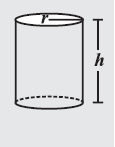<<Back to NCERT / CBSE Notes